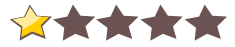# Maths LibraryFor: Star Trek: Bridge Commander
Size: 1.29 MB
 Rate this patch:Description
Functions Available:
pow(x,y) finds x^y you can also use sqed(x) or cbed(x)
root(x,y) y finds y√x you can also use sqrt(x) or cbrt(x)
log(x,y) finds log_y x you can also use ln(x) or log10(x)
frac(x) finds x!
Trig Functions are: sin(x), cos(x), tan(x) and inverse functions are: Isin(x), Icos(x) Itan(x) Note a r in front is used to for use with radians the format BC uses by default.
dtr(x) and rtd(x) convent between degrees and radians and visa verse
CheckBits(x,n) returns the state on the nth bit of x
SwapBit(x,n) changes the state
TurnOnBit(x,n) sets it to 1
TurnOffBit(x,n) sets it to 0
GetBits(x,n1,n2) returns the value in the bits n1 to n2
SetBits(x,n1,n2,y) set the bitsn1 to n2 to the value of y

ChangesVersion 1.1
- The pow(x,y) command now attempts the build in function, which I found out about, if it fails, it uses my version, this will improve speed, for people how BC has the function working. I’m leaving my one in until, I can be absolute sure of why I’ve had errors when try to use the build in function in the past.
- Add support for inverse tan the command is Itan(x), and rItan(x), which I had been able to work out when I made the previous release.
No comments. Comment to start the discussion!